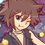# Number Theory (Thailand Math POSN 3rd round)

Not so much interesting problems there, and I'm not allowed to take exams back.

Denote $\displaystyle \left(\frac{a}{n}\right)$ as Legendre symbol.

Def: Order of $a$ modulo $n$ denoted as $ord_{n}(a)$ is the smallest positive integer $k$ such that

$a^{k} \equiv 1 \pmod{n}$

Def: $\mathbb{Z}_{n} = \{0,1,2,\dots,n-1\}$ be complete residue system modulo $n$.

Def: $\mathbb{Z}_{n}^{*} = \{x \in \mathbb{Z}_{n} | (x,n) = 1\}$ be reduced residue system modulo $n$.

1.) Find all positive integers $n$ such that

• $2 \leq n \leq 30$
• For all $x \in \mathbb{Z}_{n}^{*}$, $ord_{n}(x) < \phi(n)$.

2.) Let $a \in \mathbb{Z}_{p}^{*}$ , $p$ be prime numbers. Prove that for all $p \geq 17$,

2.1) If $\displaystyle \left(\frac{a}{p}\right) = 1$, then $ord_{p}(a) < p-1$

2.2) There exists $a$ such that $\displaystyle \left(\frac{a}{p}\right) = -1$ and $ord_{p}(a) < p-1$

3.) Let $p$ be primes from $2$ to $97$ inclusively. Find the number of primes such that

3.1) $ord_{p}(2) = p-1$

3.2) $\displaystyle \left(\frac{-1}{p}\right) = 1$Note by Samuraiwarm Tsunayoshi
6 years, 3 months ago

This discussion board is a place to discuss our Daily Challenges and the math and science related to those challenges. Explanations are more than just a solution — they should explain the steps and thinking strategies that you used to obtain the solution. Comments should further the discussion of math and science.

When posting on Brilliant:

• Use the emojis to react to an explanation, whether you're congratulating a job well done , or just really confused .
• Ask specific questions about the challenge or the steps in somebody's explanation. Well-posed questions can add a lot to the discussion, but posting "I don't understand!" doesn't help anyone.
• Try to contribute something new to the discussion, whether it is an extension, generalization or other idea related to the challenge.

MarkdownAppears as
*italics* or _italics_ italics
**bold** or __bold__ bold
- bulleted- list
• bulleted
• list
1. numbered2. list
1. numbered
2. list
Note: you must add a full line of space before and after lists for them to show up correctly
paragraph 1paragraph 2

paragraph 1

paragraph 2

[example link](https://brilliant.org)example link
> This is a quote
This is a quote
    # I indented these lines
# 4 spaces, and now they show
# up as a code block.

print "hello world"
# I indented these lines
# 4 spaces, and now they show
# up as a code block.

print "hello world"
MathAppears as
Remember to wrap math in $$ ... $$ or $ ... $ to ensure proper formatting.
2 \times 3 $2 \times 3$
2^{34} $2^{34}$
a_{i-1} $a_{i-1}$
\frac{2}{3} $\frac{2}{3}$
\sqrt{2} $\sqrt{2}$
\sum_{i=1}^3 $\sum_{i=1}^3$
\sin \theta $\sin \theta$
\boxed{123} $\boxed{123}$